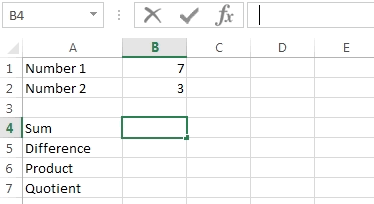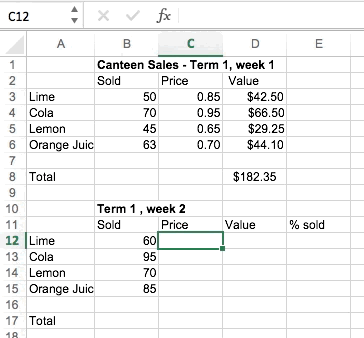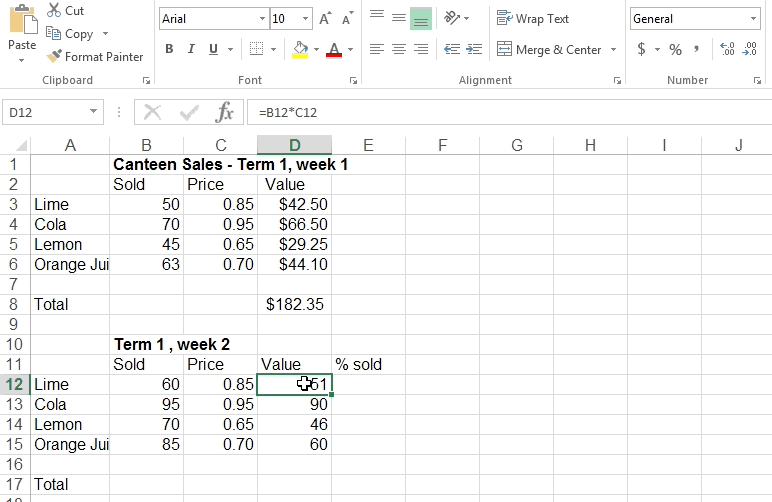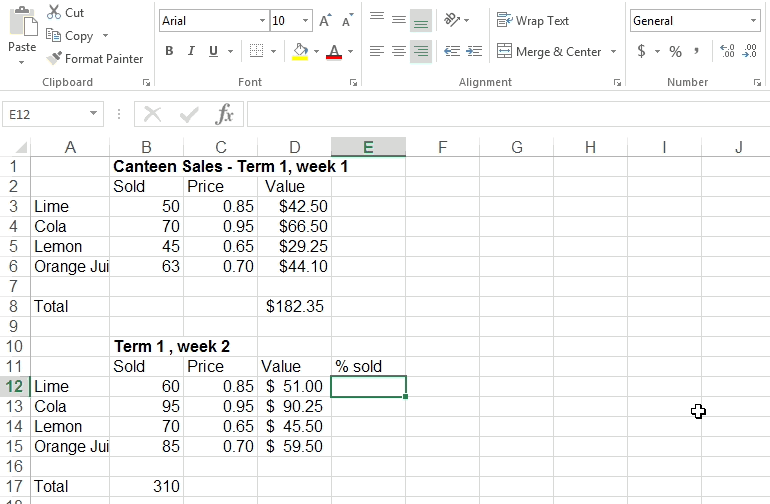# Mr Job's Maths Class

## Lessons

Topics:

### Changing the look

1. Centre the numbers in columns B, C and D.
2. Move the position of the 'Total' label.
3. Make the column A wider to fit the 'Orange Juice' label.

### Entering Formulas

Formulas are entered into spreadsheets by starting with an = sign.

The five basic mathematical operations used in formulas are:

`+` addition
`-` subtraction
`*` multiplication (* is shift 8 on the keyboard)
`/` division (bottom row of keys, right-hand side)
`^` power (shift 6)Addition: `=B1+B2`

Subtraction: `=B1-B2`

Multiplication: `=B1*B2`

Division: `=B1/B2`

### Canteen

Use the Canteen Sales spreadsheet from above.

1. Enter the week 1 prices into column C for week 2.
2.
• Enter a formula into cell `D12` that multiplies the values in cells `B12` and `C12`.
• What amount is showing now in cell `D12`?
• What does the amount in cell `D12` represent?
3. The formula in cell `D12` multiplies the number of drinks sold by the price per drink. The same formula is required in cells `D13` to `D15`.

To put this formula into cells `D13` to `D15` use Fill Down (see picture).4. Find the total number of drinks sold in Week 2.

• Click on cell `B17`.

• Type `=SUM(B12:B15)` and press Enter.

• How many drinks were sold at the canteen during week 2?

5. Enter a formula in cell `D17` to calculate the total value of the drinks sold in week 2.

### Formatting cells

Format cells `D12` to `D17` to be currency.### Calculating Percentages

Complete the steps in the image to calculate the percentage of each drink sold compared with the total number of drinks sold.Wolfram Alpha can do many maths questions.

Scientific Calculator